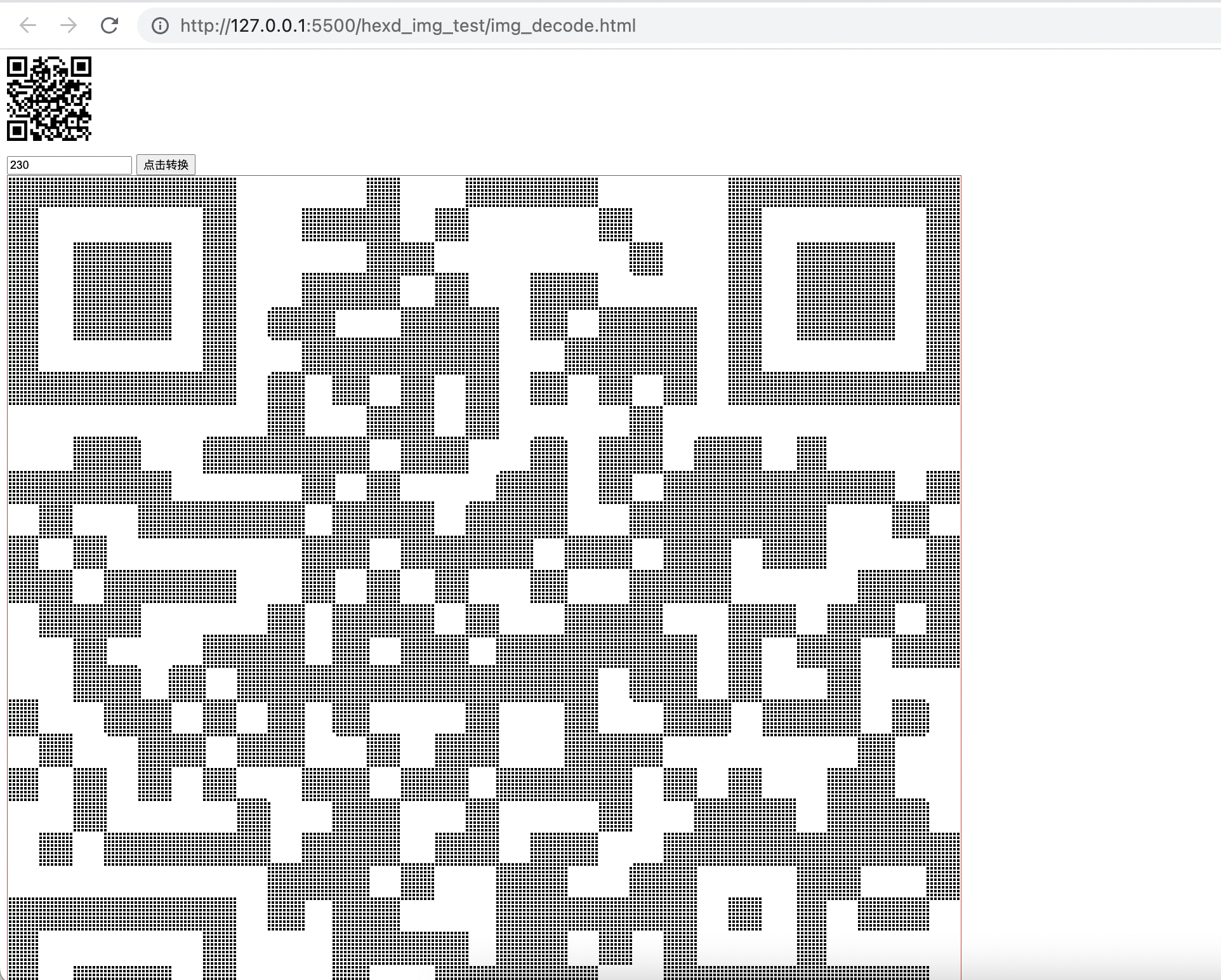«

# 寻找可靠的长久的存储介质之旅，以及背后制作的三个网页

base64 与文件互转工具：https://www.ccgxk.com/124.html<style>
* {
box-sizing: border-box
}
table {
border: red 1px solid;
}
td {
border: 0;
width: 3px;
height: 3px;
}
.the_black{
background: black;
}
</style>

<body>
<p class="image">
<img src="./img08.png" width="100px"  id="img" />
</p>
<input type="number" value="230" id="blackThreshold" />
<button onclick="drawTableImg()">点击转换</button>
<canvas id="myCanvas" width="250"  style="display: none;"></canvas>
<div id="tableDiv"></div>
<body>

<script>
const dom = document.getElementById("myCanvas"); // canvas画布
let colorData = new Array;
let canvasWidth = dom.width;  // 获取 canvas 元素上的宽度，以在转 2 维数组时作为分行依据
let imgDom = document.getElementById("img");

function  drawTableImg(){
let threshold = document.getElementById("blackThreshold").value;  // 获取黑色阈值（0~255）
getImageData(dom, imgDom.src).then((data)=>{
let colorData = data;
let colorData2d = dataTo2d(colorData, canvasWidth, threshold);  // 一维转单色、二维
// 绘制
outTable(colorData2d);
})
}

/**
* 获取图片源像素信息
*/
function getImageData(dom, url){
const ctx = dom.getContext("2d");   // 设置在画布上绘图的环境
const image = new Image();
image.src = url;

let imgH = document.getElementById("img").height;
dom.height = dom.width * (imgH/100);
//获取画布宽高
const w = dom.width;
const h = dom.height ;
return new Promise((resolve)=>{
ctx.drawImage(image, 0, 0 ,w,h);                           // 将图片绘制到画布上
const imgData = ctx.getImageData(0,0,w,h);    // 获取画布上的图像像素
resolve(imgData.data)  // 获取到的数据为一维数组,包含图像的 RGBA四个通道数据
ctx.clearRect(0,0,w,h);
}
})
}

/**
* 把颜色数组改成一维数组
*/
function getColor(array, threshold){
let result = new Array();
let nCutTimes = array.length / 4;
for (let index = 0; index < nCutTimes; index++) {
let key = array[index * 4];
result[index] = (key > threshold) ? 0 : 1;
}
return result;
}

/**
* 改成二维数组（图片平铺）
* @param array 数组（颜色数据）
* @param width 图宽（宽度像素值）
*/
function to2dArray(array, width){
let arrLen = array.length;
let result = new Array();
result = new Array();  // 初始化第一行

for (let index = 0,key = 0,line = 0; index < arrLen; index++) {
out("index" + index + "   key" + key + "   line" + line);
result[line][key] = array[index];
if(key === (width - 1)){  // 如果到每一行的最后一个元素了，就另起一行（申请新数组，行号变量加一，key 清零）
line++;
if(width * (line+1) > arrLen) break;  // 如果新的一行大于图像的高度，则退出循环
result[line] = new Array();
key = 0;
}else{
key++
}
}
return result;
}

/**
* 渲染输出成黑白表格
*/
function outTable(array2d){
out(array2d);
let outTableData = <table>;
for(let i = 0 ; i < array2d.length; i++){
outTableData += </tr>;
for(let j = 0 ; j < array2d[i].length; j++){
if(array2d[i][j] == 1){
outTableData += <td class="the_black"></td>;
}else{
outTableData += <td></td>;
}

}
outTableData += </tr>;
}
outTableData += </table>;  // 渲染完毕

document.getElementById("tableDiv").innerHTML = outTableData;  // 输出
}

/**
* 将图片源代码数据转化为二维数组
*/
function dataTo2d(array, width, threshold){
array = getColor(array, threshold);
array = to2dArray(array, width);
return array;
}